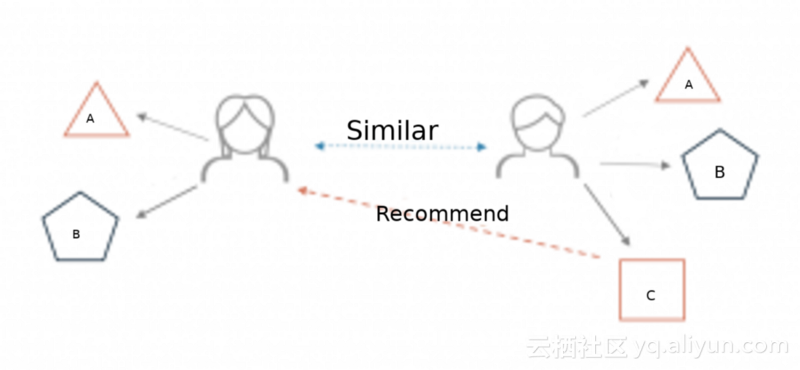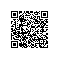# 利用python构建一个简单的推荐系统

## 什么是推荐系统？## 推荐系统的主要分类

1. 基于用户的协同过滤（User-based collaborative filtering）：主要考虑的是用户和用户之间的相似度，只要找出相似用户喜欢的物品，并预测目标用户对对应物品的评分，就可以找到评分最高的若干个物品推荐给用户。举个例子，Derrick和Dennis拥有相似的电影喜好，当新电影上映后，Derick对其表示喜欢，那么就能将这部电影推荐给Dennis。
2. 基于项目的协同过滤（Item-based collaborative filtering）：主要考虑的是物品和物品之间的相似度，只有找到了目标用户对某些物品的评分，那么就可以对相似度高的类似物品进行预测，将评分最高的若干个相似物品推荐给用户。举个例子，如果用户A、B、C给书籍X,Y的评分都是5分，当用户D想要买Y书籍的时候，系统会为他推荐X书籍，因为基于用户A、B、C的评分，系统会认为喜欢Y书籍的人在很大程度上会喜欢X书籍。

## 数据集

import pandas as pd
import numpy as np
import warnings
warnings.filterwarnings('ignore')

df = pd.read_csv('u.data', sep='\t',names=['user_id','item_id','rating','titmestamp'])

df.head()

movie_titles = pd.read_csv('Movie_Titles')
movie_titles.head()

df = pd.merge(df, movie_titles, on='item_id')
df.head()

User_id：用户ID
Item_id：电影ID
Rating：用户给电影的评分，介于1到5分之间
Timestamp：对电影进行评分的时间点
Title：电影标题

df.describe()

ratings = pd.DataFrame(df.groupby('title')['rating'].mean())
ratings.head()

ratings['number_of_ratings'] = df.groupby('title')['rating'].count()
ratings.head()

import matplotlib.pyplot as plt
%matplotlib inline
ratings['rating'].hist(bins=50)

ratings['number_of_ratings'].hist(bins=60)

import seaborn as sns
sns.jointplot(x='rating', y='number_of_ratings', data=ratings)

movie_matrix = df.pivot_table(index='user_id', columns='title', values='rating')
movie_matrix.head()

ratings.sort_values('number_of_ratings', ascending=False).head(10)

AFO_user_rating = movie_matrix['Air Force One (1997)']
contact_user_rating = movie_matrix['Contact (1997)']

Dataframe中包含user_id和对应用户给这两个电影的评分。利用如下代码进行查看。

AFO_user_rating.head()
contact_user_rating.head()

similar_to_air_force_one=movie_matrix.corrwith(AFO_user_rating)

similar_to_air_force_one.head()

similar_to_contact = movie_matrix.corrwith(contact_user_rating)

similar_to_contact.head()

corr_contact = pd.DataFrame(similar_to_contact, columns=['Correlation'])
corr_contact.dropna(inplace=True)
corr_AFO = pd.DataFrame(similar_to_air_force_one, columns=['correlation'])
corr_AFO.dropna(inplace=True)
corr_AFO.head()

corr_AFO = corr_AFO.join(ratings['number_of_ratings'])
corr_contact = corr_contact.join(ratings['number_of_ratings'])
corr_contact.head()

corr_AFO[corr_AFO['number_of_ratings'] > 100].sort_values(by='correlation', ascending=False).head(10)

corr_contact[corr_contact['number_of_ratings'] > 100].sort_values(by='Correlation', ascending=False).head(10)

《超时空接触》最相似的电影是《费城》，相关系数为0.446，被评分次数为137。根据此结果，我们可以向喜欢《超时空接触》的用户推荐列表中的电影。

## 改进使用钉钉扫一扫加入圈子
+ 订阅